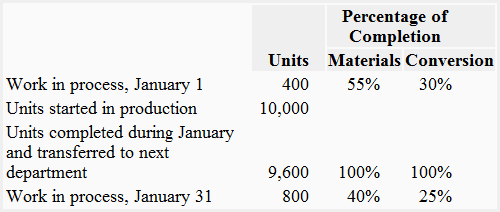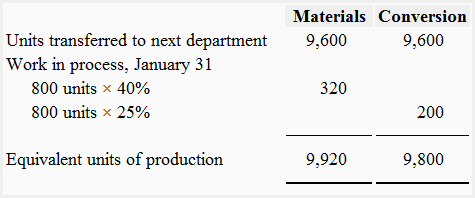# Equivalent units of production – weighted average method

## Definition and explanation of the concept of equivalent units

In process costing system, the equivalent units may be defined as the partially complete units expressed in terms of fully complete units.

The processing departments often have partially complete units in ending inventory known as work in process ending inventory. In order to compute the per unit cost of a department, we need to determine the total output of that department. As the partially complete units cannot be considered as fully complete units for the purpose of determining the department’s total output and per unit cost for a particular period, it is convenient to mathematically convert those units into a smaller number of fully complete units.

The following exhibition might be helpful to understand the concept of equivalent units:In process costing system, the equivalent number of units are obtained by multiplying the number of partially completed units by the percentage of completion of those units. The formula for computing equivalent units can be written as follows:

Equivalent units = Number of partially completed units × Percentage of completion

Suppose, for example, Department X has completed 5,000 units during the month and also has 800 units in its work in process ending inventory that are 50% complete. The 800 units in work in process ending inventory are 50% complete which means they are equivalent to 400 fully complete units as computed below:

Equivalent units = 800 units × 50%
= 400 units

In order to compute the total output of department X for the month, these equivalent units would be added to the units completed during the month. The department X’s total output for the month is, therefore, 5,400 units (5,000 units completed during the month + 400 equivalent units in ending work in process inventory).

In process costing, the total output of a department during a particular period of time is usually termed as equivalent units of production. In our example, the equivalent units of production of department X is 5,400 units which could be used for computing per unit cost of the department.

There are two methods for computing equivalent units of production – weighted average method and FIFO method. In this article, we have explained and exemplified the weighted average method. After reading this article, navigate to the next article of this chapter to read the FIFO method of computing equivalent units of production.

## Weighted average method of equivalent units of production

The weighted average method of computing equivalent units of production blends together the units and cost of current period with the units and cost of previous period. Under this method, the equivalents units of production in a department are equal to the units completed and transferred out plus the equivalent units in in ending work in process inventory.

### Formula

Under weighted average method, the equivalent units of production are computed using the following formula:

Equivalent units of production = Units transferred out + (Units in ending inventory × Percentage of completion)

## Example

The Roberts Company provides you the following data of department XRequired: Using the data of department X given above, compute equivalent units of production for the month of January using weighted average method.

#### Solution#### Explanation

1. Units transferred to next department: The units transferred to next department or finished goods store room are always assumed to be 100% complete as to materials and conversion costs. We do not need to compute equivalent units for 9,600 units transferred to the next department because they would not have been transferred out unless they were fully processed.
2. Work in process, January 31: The 800 units in work in process ending inventory have been 40% completed for materials cost and 25% completed for conversion cost during the month. In order to obtain the equivalent units for materials and conversion cost, these partially completed units have been multiplied by their percentage of completion which is 40% for materials and 25% for conversion cost.
3. Work process, January 1: The 400 units in work in process beginning inventory have been ignored while computing department X’s equivalent units of production. The reason is that the weighted average method blends together the costs and units of previous period with the costs and units of current period. It is not concerned with the fact that some work was completed in the previous period.